CGAL 4.12 - Triangulated Surface Mesh Shortest Paths
User Manual

This package provides an algorithm to compute geodesic shortest paths on a triangulated surface mesh.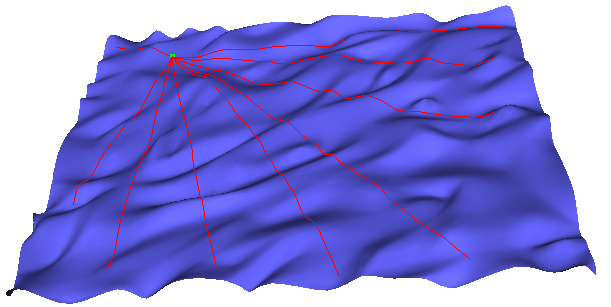Figure 66.1 Shortest paths on a terrain using one source point represented by a green square.

# Introduction

The motion planning of a robot across the surface of a 3-dimensional terrain is a typical application of the shortest path computation. Using a 2-dimensional approximation would fail to capture anything interesting about the terrain we are trying to cross, and would give a poor solution. The problem is often called the Discrete Geodesic Problem. Although the more general version of this problem, shortest paths in 3D in the presence of obstacles, is NP-Hard, when the motion is constrained to the 2D surface of an object it can be solved efficiently.

The algorithm implemented in this package builds a data structure to efficiently answer queries of the following form: Given a triangulated surface mesh $$\cal{M}$$, a set of source points $$S$$ on $$\cal{M}$$, and a target point $$t$$ also on $$\cal{M}$$, find a shortest path $$\lambda$$ between $$t$$ and any element in $$S$$, where $$\lambda$$ is constrained to the surface of $$\cal{M}$$.

The algorithm used is based on a paper by Xin and Wang , a fast and practical algorithm for exact computation of geodesic shortest paths. It is an extension of earlier results by Chen and Han  and Mitchell, Mount, and Papadimitriou  .

# User Interface Description

## Surface Mesh Shortest Path Class

The main class of this package is Surface_mesh_shortest_path. In the following we describe the typical workflow when using this class

### Specifying the Input

The shortest paths are computed on a triangulated surface mesh, represented by a model of the FaceListGraph concept. There is no restriction on the genus, connectivity, or convexity of the input surface mesh.

For efficiency reason, index property maps for vertices, halfedges and faces are internally used. For each simplex type the property map must provide an index between 0 and the number of simplices. We recommend to use the class CGAL::Surface_mesh as model of FaceListGraph. If you use the class CGAL::Polyhedron_3, you should use it with the item class CGAL::Polyhedron_items_with_id_3, for which default property maps are provided. This item class associates to each simplex an index that provides a $$O(1)$$ time access to the indices. Note that the initialization of the property maps requires a call to set_halfedgeds_items_id().

The access to the embedding of each vertex is done using a point vertex property map associating to each vertex a 3D point. Defaults are provided for CGAL classes.

If the traits class used holds some local state, it must also be passed to the class when constructing it (the default one provided does not).

### Specifying the Source Points

The set of source points for shortest path queries can be populated one by one or using a range. A source point can be specified using either a vertex of the input surface mesh or a face of the input surface mesh with some barycentric coordinates. Given a point $$p$$ that lies inside a triangle face $$(A,B,C)$$, its barycentric coordinates are a weight triple $$(b_0,b_1,b_2)$$ such that $$p = b_0\cdot~A + b_1\cdot~B + b_2\cdot~C$$, and $$b_0 + b_1 + b_2 = 1$$.

### Building the Internal Sequence Tree

A time consuming operation for shortest path queries consists in building an internal data structure used to make the queries. This data structure is called the sequence tree. It will be built automatically when the first shortest path query is done and will be reused for any subsequent query as long as the set of source points does not change. Each time the set of source points is changed the sequence tree needs to be rebuilt (if already built). Note that it can also be built manually by a call to Surface_mesh_shortest_path::build_sequence_tree().

### Shortest Path Queries

As for specifying the source points, the target point for a shortest path query can be specified using either a vertex of the input surface mesh or a face of the input surface mesh and some barycentric coordinates.

There are three different kinds of query functions that can be called using the class Surface_mesh_shortest_path. Given a target point, all these functions compute the shortest path between that target point and the set of source points:

• Surface_mesh_shortest_path::shortest_distance_to_source_points() provides the closest source point to the target point together with the length of the shortest path.
• Surface_mesh_shortest_path::shortest_path_points_to_source_points() provides all the intersection points of the shortest path with the edges and vertices of the input surface mesh (including the source and the target point). This function is useful for visualization purposes.
• Surface_mesh_shortest_path::shortest_path_sequence_to_source_points gives access to the complete sequence of simplices crossed by the shortest path using a visitor object model of the concept SurfaceMeshShortestPathVisitor.

Some convenience functions are provided to compute:

• the point on the input surface mesh specified as a face of the input surface mesh and some barycentric coordinates.
• the closest point on the input surface mesh (specified as a face of the input surface mesh and some barycentric coordinates) to a given 3D point. Those function are using the class CGAL::AABB_tree.

## Kernel Recommendations

In short, we recommend to use a CGAL kernel with exact predicates such as CGAL::Exact_predicates_inexact_constructions_kernel.

If you need the constructions to be exact (for the shortest path point computation for example), you should use a kernel with exact constructions. Although the algorithm uses square root operations, it will also work on geometry kernels which do not support them by first converting the kernel's number type to double, using the std::sqrt, and converting it back. Note that it would be preferable to use a kernel with directly supports square roots to get the most precision of the shortest path computations.

Using a kernel such as CGAL::Exact_predicates_exact_constructions_kernel_with_sqrt with this package will indeed provide the exact shortest paths, but it will be extremely slow. Indeed, in order to compute the distance along the surface, it is necessary to unfold sequences of faces, edge-to-edge, out into a common plane. The functor SurfaceMeshShortestPathTraits::Construct_triangle_3_to_triangle_2_projection provides an initial layout of the first face in a sequence, by rotating a given face into the xy-plane. SurfaceMeshShortestPathTraits::Construct_triangle_3_along_segment_2_flattening unfolds a triangle into the plane, using a specified segment as a base. Since this results in a chain of constructed triangles in the plane, the exact representation types used with this kernel (either CORE::Expr or leda_real) will process extremely slow, even on very simple inputs. This is because the exact representations will effectively add an $$O(n)$$ factor to every computation.

# Examples

## Simple Example

The following example shows how to get the shortest path to every vertex from an arbitrary source point on a surface. The shortest path class needs to have an index associated to each vertex, halfedge and face, which is naturally given for the class Surface_mesh.

#include <cstdlib>
#include <iostream>
#include <fstream>
#include <CGAL/Exact_predicates_inexact_constructions_kernel.h>
#include <CGAL/Random.h>
#include <CGAL/Surface_mesh.h>
#include <boost/lexical_cast.hpp>
typedef CGAL::Surface_mesh_shortest_path<Traits> Surface_mesh_shortest_path;
typedef boost::graph_traits<Triangle_mesh> Graph_traits;
typedef Graph_traits::vertex_iterator vertex_iterator;
typedef Graph_traits::face_iterator face_iterator;
int main(int argc, char** argv)
{
Triangle_mesh tmesh;
std::ifstream input((argc>1)?argv:"data/elephant.off");
input >> tmesh;
input.close();
// pick up a random face
const unsigned int randSeed = argc > 2 ? boost::lexical_cast<unsigned int>(argv) : 7915421;
CGAL::Random rand(randSeed);
const int target_face_index = rand.get_int(0, static_cast<int>(num_faces(tmesh)));
face_iterator face_it = faces(tmesh).first;
// ... and define a barycentric coordinates inside the face
Traits::Barycentric_coordinates face_location = {{0.25, 0.5, 0.25}};
// construct a shortest path query object and add a source point
Surface_mesh_shortest_path shortest_paths(tmesh);
// For all vertices in the tmesh, compute the points of
// the shortest path to the source point and write them
// into a file readable using the CGAL Polyhedron demo
std::ofstream output("shortest_paths_with_id.cgal");
vertex_iterator vit, vit_end;
for ( boost::tie(vit, vit_end) = vertices(tmesh);
vit != vit_end; ++vit)
{
std::vector<Traits::Point_3> points;
shortest_paths.shortest_path_points_to_source_points(*vit, std::back_inserter(points));
// print the points
output << points.size() << " ";
for (std::size_t i = 0; i < points.size(); ++i)
output << " " << points[i];
output << std::endl;
}
return 0;
}

## Example Using Polyhedron_3

The following example shows how to get the shortest path to every vertex from an arbitrary source point on the surface. Note that this example uses the Polyhedron_items_with_id_3 item class. The shortest path class needs to have an index associated to each vertex, halfedge and face. Using this item class provide an efficient direct access to the required indices.

#include <cstdlib>
#include <iostream>
#include <fstream>
#include <iterator>
#include <CGAL/Exact_predicates_inexact_constructions_kernel.h>
#include <CGAL/Random.h>
#include <CGAL/Polyhedron_3.h>
#include <CGAL/Polyhedron_items_with_id_3.h>
#include <boost/lexical_cast.hpp>
typedef CGAL::Surface_mesh_shortest_path<Traits> Surface_mesh_shortest_path;
typedef boost::graph_traits<Triangle_mesh> Graph_traits;
typedef Graph_traits::vertex_iterator vertex_iterator;
typedef Graph_traits::face_iterator face_iterator;
int main(int argc, char** argv)
{
Triangle_mesh tmesh;
std::ifstream input((argc>1)?argv:"data/elephant.off");
input >> tmesh;
input.close();
// initialize indices of vertices, halfedges and faces
// pick up a random face
const unsigned int randSeed = argc > 2 ? boost::lexical_cast<unsigned int>(argv) : 7915421;
CGAL::Random rand(randSeed);
const int target_face_index = rand.get_int(0, static_cast<int>(num_faces(tmesh)));
face_iterator face_it = faces(tmesh).first;
// ... and define a barycentric coordinates inside the face
Traits::Barycentric_coordinates face_location = {{0.25, 0.5, 0.25}};
// construct a shortest path query object and add a source point
Surface_mesh_shortest_path shortest_paths(tmesh);
// For all vertices in the tmesh, compute the points of
// the shortest path to the source point and write them
// into a file readable using the CGAL Polyhedron demo
std::ofstream output("shortest_paths_with_id.cgal");
vertex_iterator vit, vit_end;
for ( boost::tie(vit, vit_end) = vertices(tmesh);
vit != vit_end; ++vit)
{
std::vector<Traits::Point_3> points;
shortest_paths.shortest_path_points_to_source_points(*vit, std::back_inserter(points));
// print the points
output << points.size() << " ";
for (std::size_t i = 0; i < points.size(); ++i)
output << " " << points[i];
output << std::endl;
}
return 0;
}

## Example Using Polyhedron Items without IDs

Although it is better to have an index built into each simplex, you can also use a surface mesh without internal indices by using external indices. The following example shows how to proceed in this case.

#include <cstdlib>
#include <iostream>
#include <fstream>
#include <iterator>
#include <CGAL/Random.h>
#include <CGAL/Exact_predicates_inexact_constructions_kernel.h>
#include <CGAL/Polyhedron_3.h>
#include <boost/lexical_cast.hpp>
typedef CGAL::Polyhedron_3<Kernel> Triangle_mesh;
// default property maps
typedef boost::property_map<Triangle_mesh,
boost::vertex_external_index_t>::type Vertex_index_map;
typedef boost::property_map<Triangle_mesh,
CGAL::halfedge_external_index_t>::type Halfedge_index_map;
typedef boost::property_map<Triangle_mesh,
CGAL::face_external_index_t>::type Face_index_map;
Vertex_index_map,
Halfedge_index_map,
Face_index_map> Surface_mesh_shortest_path;
typedef boost::graph_traits<Triangle_mesh> Graph_traits;
typedef Graph_traits::vertex_iterator vertex_iterator;
typedef Graph_traits::halfedge_iterator halfedge_iterator;
typedef Graph_traits::face_iterator face_iterator;
int main(int argc, char** argv)
{
Triangle_mesh tmesh;
std::ifstream input((argc>1)?argv:"data/elephant.off");
input >> tmesh;
input.close();
// pick up a random face
const unsigned int randSeed = argc > 2 ? boost::lexical_cast<unsigned int>(argv) : 7915421;
CGAL::Random rand(randSeed);
const int target_face_index = rand.get_int(0, static_cast<int>(num_faces(tmesh)));
face_iterator face_it = faces(tmesh).first;
// ... and define a barycentric coordinates inside the face
Traits::Barycentric_coordinates face_location = {{0.25, 0.5, 0.25}};
// construct a shortest path query object and add a source point
// Note that the external index property map are automatically initialized
Surface_mesh_shortest_path shortest_paths(tmesh,
get(boost::vertex_external_index, tmesh),
get(CGAL::halfedge_external_index, tmesh),
get(CGAL::face_external_index, tmesh),
get(CGAL::vertex_point, tmesh));
// For all vertices in the tmesh, compute the points of
// the shortest path to the source point and write them
// into a file readable using the CGAL Polyhedron demo
std::ofstream output("shortest_paths_no_id.cgal");
vertex_iterator vit, vit_end;
for ( boost::tie(vit, vit_end) = vertices(tmesh);
vit != vit_end; ++vit)
{
std::vector<Traits::Point_3> points;
shortest_paths.shortest_path_points_to_source_points(*vit, std::back_inserter(points));
// print the points
output << points.size() << " ";
for (std::size_t i = 0; i < points.size(); ++i)
output << " " << points[i];
output << std::endl;
}
return 0;
}

## Using Multiple Source Points

This example shows how to compute the sequence tree from multiple source points, using an iterator range of Surface_mesh_shortest_path::Face_location objects generated at random.

#include <cstdlib>
#include <iostream>
#include <fstream>
#include <iterator>
#include <CGAL/Exact_predicates_inexact_constructions_kernel.h>
#include <CGAL/Random.h>
#include <CGAL/Surface_mesh.h>
typedef CGAL::Surface_mesh_shortest_path<Traits> Surface_mesh_shortest_path;
typedef boost::graph_traits<Triangle_mesh> Graph_traits;
typedef Graph_traits::vertex_iterator vertex_iterator;
typedef Graph_traits::face_iterator face_iterator;
typedef Graph_traits::face_descriptor face_descriptor;
int main(int argc, char** argv)
{
Triangle_mesh tmesh;
std::ifstream input((argc>1)?argv:"data/elephant.off");
input >> tmesh;
input.close();
// pick up some source points inside faces,
const unsigned int randSeed = argc > 2 ? boost::lexical_cast<unsigned int>(argv) : 7915421;
CGAL::Random rand(randSeed);
// by copying the faces in a vector to get a direct access to faces
std::size_t nb_faces=num_faces(tmesh);
face_iterator fit, fit_end;
boost::tie(fit, fit_end) = faces(tmesh);
std::vector<face_descriptor> face_vector(fit, fit_end);
// and creating a vector of Face_location objects
const std::size_t nb_source_points = 30;
Traits::Barycentric_coordinates face_location = {{0.25, 0.5, 0.25}};
std::vector<Face_location> faceLocations(nb_source_points, Face_location(face_descriptor(), face_location));
for (std::size_t i = 0; i < nb_source_points; ++i)
{
faceLocations[i].first=face_vector[rand.get_int(0, static_cast<int>(nb_faces))];
}
// construct a shortest path query object and add a range of source points
Surface_mesh_shortest_path shortest_paths(tmesh);
// For all vertices in the tmesh, compute the points of
// the shortest path to the source point and write them
// into a file readable using the CGAL Tmesh demo
std::ofstream output("shortest_paths_multiple_sources.cgal");
vertex_iterator vit, vit_end;
for ( boost::tie(vit, vit_end) = vertices(tmesh);
vit != vit_end; ++vit)
{
std::vector<Traits::Point_3> points;
shortest_paths.shortest_path_points_to_source_points(*vit, std::back_inserter(points));
// print the points
output << points.size() << " ";
for (std::size_t i = 0; i < points.size(); ++i)
output << " " << points[i];
output << std::endl;
}
return 0;
}

## Shortest Path Sequence Visitor

This example shows how to implement a model of the SurfaceMeshShortestPathVisitor concept to get detailed information about the sequence of simplicies crossed by a shortest path.

#include <cstdlib>
#include <iostream>
#include <fstream>
#include <CGAL/Exact_predicates_inexact_constructions_kernel.h>
#include <CGAL/Random.h>
#include <CGAL/Surface_mesh.h>
#include <boost/variant.hpp>
#include <boost/lexical_cast.hpp>
typedef CGAL::Surface_mesh_shortest_path<Traits> Surface_mesh_shortest_path;
typedef Traits::Barycentric_coordinates Barycentric_coordinates;
typedef boost::graph_traits<Triangle_mesh> Graph_traits;
typedef Graph_traits::vertex_iterator vertex_iterator;
typedef Graph_traits::face_iterator face_iterator;
typedef Graph_traits::vertex_descriptor vertex_descriptor;
typedef Graph_traits::face_descriptor face_descriptor;
typedef Graph_traits::halfedge_descriptor halfedge_descriptor;
// A model of SurfacemeshShortestPathVisitor storing simplicies
// using boost::variant
struct Sequence_collector
{
typedef boost::variant< vertex_descriptor,
std::pair<halfedge_descriptor,double>,
std::pair<face_descriptor, Barycentric_coordinates> > Simplex;
std::vector< Simplex > sequence;
void operator()(halfedge_descriptor he, double alpha)
{
sequence.push_back( std::make_pair(he, alpha) );
}
void operator()(vertex_descriptor v)
{
sequence.push_back( v );
}
void operator()(face_descriptor f, Barycentric_coordinates alpha)
{
sequence.push_back( std::make_pair(f, alpha) );
}
};
// A visitor to print what a variant contains using boost::apply_visitor
struct Print_visitor : public boost::static_visitor<> {
int i;
Triangle_mesh& g;
Print_visitor(Triangle_mesh& g) :i(-1), g(g) {}
void operator()(vertex_descriptor v)
{
std::cout << "#" << ++i << " : Vertex : " << get(boost::vertex_index, g)[v] << "\n";
}
void operator()(const std::pair<halfedge_descriptor,double>& h_a)
{
std::cout << "#" << ++i << " : Edge : " << get(CGAL::halfedge_index, g)[h_a.first] << " , ("
<< 1.0 - h_a.second << " , "
<< h_a.second << ")\n";
}
void operator()(const std::pair<face_descriptor, Barycentric_coordinates>& f_bc)
{
std::cout << "#" << ++i << " : Face : " << get(CGAL::face_index, g)[f_bc.first] << " , ("
<< f_bc.second << " , "
<< f_bc.second << " , "
<< f_bc.second << ")\n";
}
};
int main(int argc, char** argv)
{
Triangle_mesh tmesh;
std::ifstream input((argc>1)?argv:"data/elephant.off");
input >> tmesh;
input.close();
// pick up a random face
const unsigned int randSeed = argc > 2 ? boost::lexical_cast<unsigned int>(argv) : 7915421;
CGAL::Random rand(randSeed);
const int target_face_index = rand.get_int(0, static_cast<int>(num_faces(tmesh)));
face_iterator face_it = faces(tmesh).first;
// ... and define a barycentric coordinates inside the face
Barycentric_coordinates face_location = {{0.25, 0.5, 0.25}};
// construct a shortest path query object and add a source point
Surface_mesh_shortest_path shortest_paths(tmesh);
// pick a random target point inside a face
face_it = faces(tmesh).first;
// collect the sequence of simplicies crossed by the shortest path
Sequence_collector sequence_collector;
shortest_paths.shortest_path_sequence_to_source_points(*face_it, face_location, sequence_collector);
// print the sequence using the visitor pattern
Print_visitor print_visitor(tmesh);
for (size_t i = 0; i < sequence_collector.sequence.size(); ++i)
boost::apply_visitor(print_visitor, sequence_collector.sequence[i]);
return 0;
}

# Benchmarks

These benchmarks were run using randomly generated source and destination points over multiple trials. The measurements were executed using CGAL 4.5, under Cygwin 1.7.32, using the Gnu C++ compiler version 4.8.3 with options -O3 -DNDEBUG. The system used was a 64bit Intel Core i3 2.20GHz processor with 6GB of RAM

## Single Source Point

Model Number of Vertices Average Construction Time (s) Average Queries Per Second Peak Memory Usage (MB)
ellipsoid.off 162 0.00258805 1.21972e+06 0.39548
anchor.off 519 0.0580262 230461 3.88799
rotor.off 600 0.0386633 326175 3.10571
spool.off 649 0.0418305 299766 3.75773
handle.off 1165 0.0976167 227343 7.66706
couplingdown.off 1841 0.138467 246833 10.1731
bones.off 2154 0.0101125 1.31834e+06 0.865896
mushroom.off 2337 0.206034 202582 22.5804
elephant.off 2775 0.136177 313785 14.0987
cow.off 2904 0.259104 206515 17.4796
knot1.off 3200 0.279455 207084 25.314
retinal.off 3643 0.255788 247617 29.8031
femur.off 3897 0.25332 264825 21.4806
knot2.off 5760 0.295655 309593 22.5549
bull.off 6200 0.513506 209994 34.983
fandisk.off 6475 0.609507 198768 71.3617
turbine.off 9210 2.23755 93079.5 172.072
man.off 17495 1.59015 187519 148.358

## Ten Source Points

Model Number of Vertices Average Construction Time (s) Average Queries Per Second Peak Memory Usage (MB)
ellipsoid.off 162 0.00321017 911025 0.245674
anchor.off 519 0.03601 353062 3.19274
rotor.off 600 0.015864 805416 1.97554
spool.off 649 0.0165743 802701 2.09675
handle.off 1165 0.0294564 646057 4.62122
couplingdown.off 1841 0.126045 272465 7.80517
bones.off 2154 0.055434 536646 4.0203
mushroom.off 2337 0.139285 290425 11.462
elephant.off 2775 0.167269 285076 11.2743
cow.off 2904 0.15432 328549 13.0676
knot1.off 3200 0.114051 454640 16.1735
retinal.off 3643 0.233208 287869 18.6274
femur.off 3897 0.128097 457112 16.8295
knot2.off 5760 0.413548 260195 33.484
bull.off 6200 0.371713 297560 30.522
fandisk.off 6475 0.545929 223865 39.5607
turbine.off 9210 1.35703 157301 90.7139
man.off 17495 1.75936 185194 122.541

## Comparison of Construction and Query Times with Multiple Source Points

The following figures track the construction time, query time, and peak memory usage for the various test models as the number of source points increases. Notice that none of the values increases significantly as the number of source points increases. In fact, in most cases, the running time and memory go down. This is because a larger number of source points tends to result in a more flat sequence tree, which translates to reduced runtime and memory costs.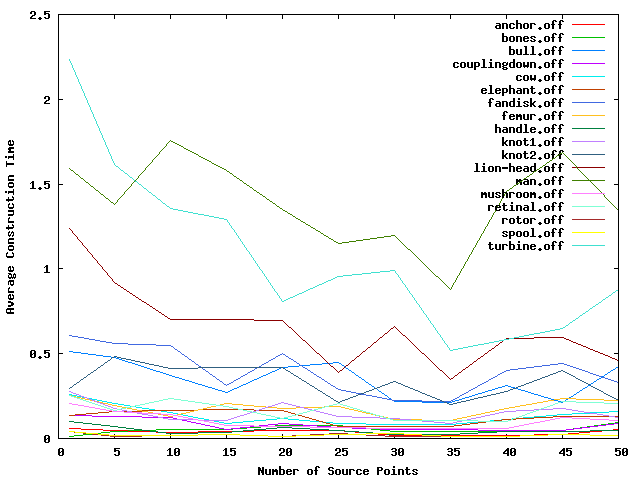Figure 66.2 Plot of construction times against different numbers of source points.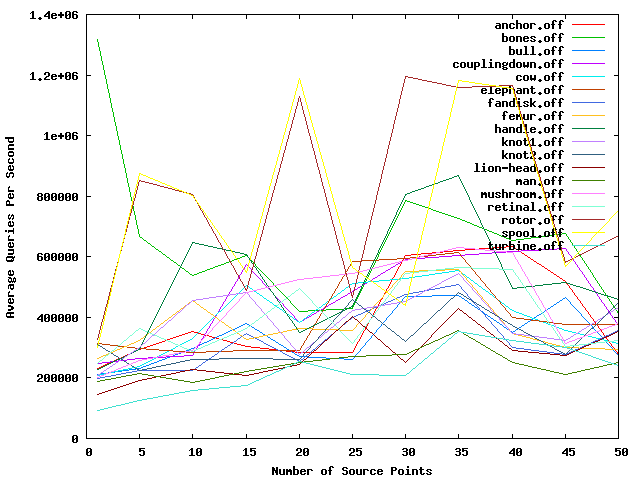Figure 66.3 Plot of query times against different numbers of source points.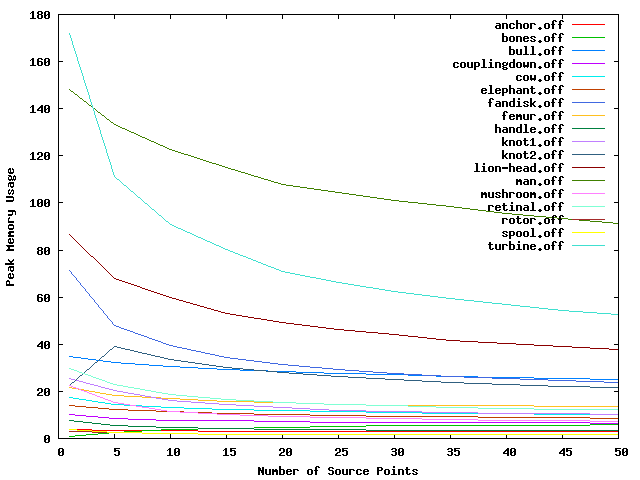Figure 66.4 Plot of peak memory usage against different numbers of source points.

# Implementation Details

## Definitions

### Geodesic Paths

A geodesic curve is a locally shortest path on the surface of some manifold, that is, it cannot be made shorter by some local perturbations. On a surface mesh, this translates to a curve where, when the faces crossed by the curve are unfolded into the plane, the curve forms a straight line. Another way of describing it is that there is exactly $$\pi$$ surface angle to both sides at every point along the curve (except possibly at the curve's endpoints).

A geodesic curve between two points is not necessarily a shortest path, but all shortest paths on surface meshes are formed by sequences of one or more geodesic paths, whose junction points are either vertices on the boundary of the mesh, or saddle vertices. We call such a curve on the surface of the mesh a potential shortest path between its two endpoints.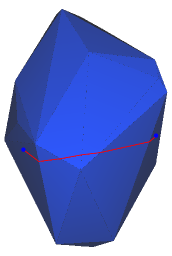Figure 66.5 A geodesic on the surface of a simple surface mesh.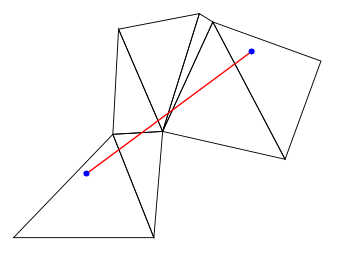Figure 66.6 The same geodesic, with its faces unfolded into the plane. Note in the unfolding, the geodesic forms a straight line.

### Visibility Window

A visibility window (or visibility cone) is a pair of geodesic curves which share a common source point and enclose a locally flat region of the surface mesh. Locally flat means that between every pair of points inside the window, there is exactly one geodesic path between them which also stays inside the bounds of the window. Thus, operations, such as distance calculations, can be done with normal 2D Euclidean operations while inside the window. When a visibility window encounters a vertex (a non-flat part of the surface), a branch occurs, forming a sub-window to either side.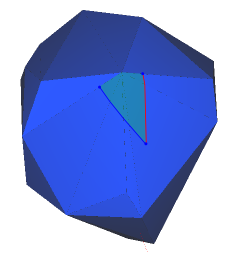Figure 66.7 A single visibility window, before it encounters a vertex.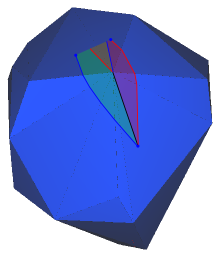Figure 66.8 After encountering a convex vertex, the visibility window branches to either side (blue on the left, red on the right). Note that the two new windows immediately overlap on the other side of the vertex, since the surrounding surface area is less than $$2 \pi$$. Points inside this region of overlap might have two possible shortest paths from the origin point.

A saddle vertex on a surface mesh is a vertex $$v$$ where the sum of surface angles of all faces incident at $$v$$ is greater than $$2 \pi$$, or, in simpler terms, one cannot flatten all the faces incident to $$v$$ into the plane without overlap. Identifying and dealing with saddle vertices are important in shortest path algorithms because they form blind spots which cannot be reached by a single geodesic curve.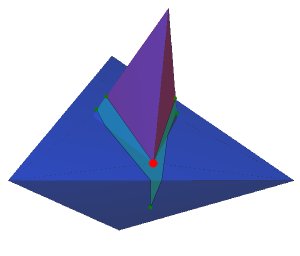Figure 66.9 A visibility window (shaded blue) encounters a saddle vertex; the shaded red region behind the vertex is not reachable by a geodesic curve from the source point (assuming the geodesic must stay inside the initial window).

In order to deal with this, we must create a new set of child visibility windows which branch out around the saddle vertex. The paths through these child windows would first arrive at the saddle vertex, and then follow a new visibility window (forming a kind of poly-line on the surface). Note that similar behavior is required when we reach a boundary vertex of a non-closed surface mesh.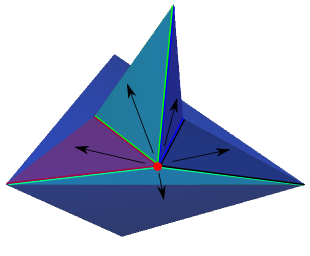Figure 66.10 In order to see past the blind spot created by the saddle vertex, we create a branching set of visibility windows emanating from the saddle vertex. Note that only the branches which cover the blind spot for the parent visibility window are needed for our algorithm.

### The Sequence Tree

In order to compute shortest paths, we build a sequence tree (or cone tree) from each source point. The sequence tree describes the combinatoric structure of all potential shortest paths which originate from a single source point, by organizing them into a hierarchy of visibility windows.

Whenever a vertex of the surface mesh is encountered, a branch occurs in the sequence tree. If the vertex is a non-saddle vertex, then only two children are created, one for each edge incident to that vertex on the current face. If the vertex is a saddle vertex, in addition to the two children mentioned above, a special type of node, called a pseudo-source, is created which branches out from that vertex.

Once a sequence tree is built, the potential shortest paths from the source to every point inside a given visibility window can be computed. The sequence of faces along each branch of the tree are laid out edge to edge, into a common plane, such that the geodesic distance from any point on the surface to its nearest source point can be obtained using a single 2D Euclidean distance computation. Note that if the window belongs to a pseudo-source, the distance is measured from the target to the pseudo-source, and then the distance from the pseudo-source back to its parent is measured, and so on back to the original source.

## Algorithm Overview

The size of the sequence tree from any source point is theoretically infinite, however we only ever care about trees which are of depth at most N, where N is the number of faces in the surface mesh (since no shortest path can cross the same face twice). Even then, the size of this truncated sequence tree is potentially exponential in the size of the surface mesh, thus a simple breadth-first search is not feasible. Rather, we apply techniques to eliminate entire branches which are provably unable to contain shortest paths from the source point(s). The techniques used are given in greater detail in a paper by Xin and Wang , which itself expands an earlier work by Chen and Han  and Mitchell, Mount, and Papadimitriou  .

Handling multiple source points is simply a matter of constructing multiple sequence trees concurrently, using a method similar to the multi-source Dijsktra's algorithm.

## Continuous Dijkstra

Continuous Dijkstra is simply the application of the graph-search algorithm to a non-discrete setting. As we build the search tree, newly created nodes are tagged with a distance metric, and inserted into a priority queue, such that the shortest distance nodes are always first.

## One Angle, One Split

This observation by Chen and Han states that out of all the branches that occur at any given vertex of the surface mesh, only a limited number have more than one child which can define shortest paths. This is accomplished by maintaining, for each vertex, all nodes of the sequence tree which can contain that vertex inside their visibility window.

• For each vertex, only one two-way branch may occur per face incident to that vertex, specifically, that of the nearest node to that vertex which crosses that face. We call that closest node the occupier of that vertex.
• If the vertex is a saddle vertex, only one pseudo-source may be established at that vertex, this time by the absolute nearest node to that vertex.

This method alone can decrease the running time for construction of the sequence tree construction to polynomial time.

## Distance Filtering

An additional distance filter proposed by Xin and Wang helps prune the search tree even further by comparing the current node's distance to the closest distance so far of the three vertices on the current face. Details on this method can be found in their paper .

## Locating Shortest Paths

In order to locate the shortest path from a target point to a source point, we must select the correct visibility window. A simple method is to keep track for each face $$f$$ of all windows which cross $$f$$. In practice, at most a constant number of windows will cross any given face, so for simplicity this is the method we employ. An alternative is to construct a Voronoi-like structure on each face, where each cell represents a visibility window. We did not attempt this method, however it would seem likely that it would be of no computational benefit.

## Pseudo-Code

In this section we give a brief outline of the pseudo-code for this algorithm. More details can be found in  and .

--
-- Global Values
--
G : FaceGraph(V,E,F)
-- V - the set of vertices
-- E - the set of edges
-- F - the set of planar faces

Q : PriorityQueue
-- A priority queue ordered using the metric given by Xin and Wang

--
-- Types
--
type VisbilityWindow:
f : a face of F, the current face of this window, we say this window 'crosses' face f
s : a point on the surface of F, the source point of this window
d :  the 'base distance' to s, only non-zero if s is a pseudo-source
l : the left-side bounding ray of this window, with its origin at s
r : the right-side bounding ray of this window, with its origin at s
p : its parent VisibilityWindow

--
-- Methods
--
method XinWangDistanceFilter:
Input:
w : a VisibilityWindow
Output:
filter : true if w passes the distance filtering metric given by Xin and Wang, false otherwise

method PropagateWindow:
Input:
w : a visibility window
e : an edge on face w.f
Output:
w' : A new visibility window on the face opposite w.f across edge e
Begin:
Let f' be the face on the opposite side of e as w.f
Lay out face f' along e, such that it shares a common plane with w.f
Create a new VisibilityWindow w', with
- w its parent
- the same source point and base distance as w
- its boundary rays clipped to the sub-segment of e covered by w
return w'

method CreateFaceWindow:
Input:
f : a face of F
v : a vertex of f
w : a VisibilityWindow which intersects f and contains v
Output:
w' : a new VisibilityWindow, with
-- w its parent
-- its source point s = v
-- its two bounding rays along the edges incident to v
-- face f as its crossed face
-- its base distance being the distance of window w to v

method CreatePseudoSource:
Input:
w : the parent window
v : a saddle vertex of V
Begin:
For each face f incident to v:
w' = CreateFaceWindow(f, v, w)
Q.insert(w')

method TreeDepth:
Input:
w : a VisibilityWindow in some sequence tree T
Output:
The depth of node w in its current sequence tree (this would typically be cached in w itself)

method ShortestPathTree:
Input:
s[1..n] : a set of source points on the surface of G.
For simplicity (and without loss of generality),
we will assume they are all vertices of G.
Output:
T[1..n] : a set of sequence trees for the source points
Declare:
O : a map of (f,v) => VisibilityWindow, which gives the 'vertex occupier' for (f,v),
that is the window which crosses face f and whose source is nearest to vertex v
S : a map of v => VisibilityWindow, which gives the window whose source is nearest
to v. Note that this is a strict subset of O
Begin:
for i in 1..n:
Let r[i] be the root of T[i], with distance 0 to s[i]
CreatePseudoSource( r[i], s[i] )
While Q is not empty:
w = Q.take()
if XinWangDistanceFilter(w) and TreeDepth(w) <= |F|:
if w contains a vertex v of w.f:
if w is closer to v than O[w.f,v]:
O[w.f,v] = w
if v is a boundary vertex or a saddle vertex, and w is closer to v than S[v]:
S[v] = w
CreatePseudoSource(w, v, w.dist(v))
let {e_0, e_1} be the edges of f incident to v
for i in [0,1]:
w' = PropagateWindow(w, e_i)
Q.insert(w')
else:
let e_n be the edge 'closer' to window w
w' = PropagateWindow(w, e_n)
Q.insert(w')
else:
let e_o be the only edge crossed by window w
w' = PropagateWindow(w, e_o)
Q.insert(w')
return T[1..n]


To perform shortest path distance queries to each vertex, we can simply use the results stored in S after the completion of ShortestPathTree, as it contains a map from each vertex to the VisibilityWindow which has the shortest path to that vertex. Performing shortest path computations to any arbitrary face location is slightly more complex. As eluded to above, after completion of the algorithm, we traverse each of the sequence trees, and for each face f we store all the VisibilityWindows which cross f in a look-up structure. Then, to find the shortest path to a point on the surface of face f, we look up that pre-stored set of VisibilityWindows associated with it, and among those windows we select the one which contains the query point and has the shortest path back to the origin. Though it may seem slow since it involves a linear search but it is efficient in practice since the number of faces crossing any single face is typically limited (this is due to the additional filtering method given by Xin and Wang .

The actual surface paths can be reconstructed by backtracking from the VisibilityWindow, through its parents in the tree up to the root, and keeping track of each face that was crossed.

# Design and Implementation History

This package is the result of the work of Stephen Kiazyk during the 2014 season of the Google Summer of Code. He has been mentored by Sébastien Loriot and Éric Colin de Verdière who also contributed to the documentation and the API definition.